# Volume and surface area - Quantitative Aptitude (MCQ) questions

Dear Readers, Welcome to Quantitative Aptitude Volume and surface area questions and answers with explanation. These Volume and surface area solved examples with shortcuts and tricks will help you learn and practice for your Placement Test and competitive exams like Bank PO, IBPS PO, SBI PO, RRB PO, RBI Assistant, LIC,SSC, MBA - MAT, XAT, CAT, NMAT, UPSC, NET etc.

After practicing these tricky Volume and surface area multiple choice questions, you will be exam ready to deal with any objective type questions.

1)   Dean has a cardboard box whose length, breadth and height are in the ratio 1:2:3. He makes a new box such that the length, breadth and height got increased by 100%, 200% and 200% respectively. How much less is volume of old box than the new box?
- Published on 03 May 17

a. 12 times less
b. 16 times less
c. 17 times less
d. 24 times less
 Answer  Explanation ANSWER: 17 times less Explanation: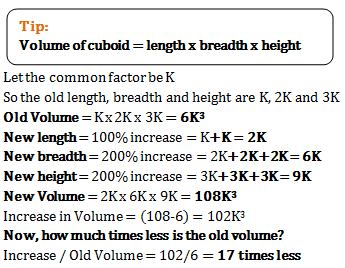2)   When Jaya divided surface area of a sphere by the sphere’s volume, she got the answer as 1/18 cm. What is the radius of the sphere?
- Published on 03 May 17

a. 24 cm
b. 6 cm
c. 54 cm
d. 4.5 cm
 Answer  Explanation ANSWER: 54 cm Explanation: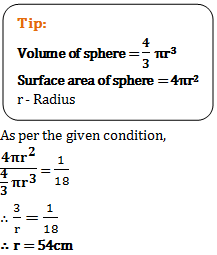3)   Ramesh has a metal cube. He paints all sides of the cube with green color. He divides the cube into smaller cubes of volume 1 cu.m. each. How many of these smaller cubes will not have green color on even one of its sides if the volume of larger cube is 27 cu.m.?
- Published on 03 May 17

a. 9
b. 1
c. 0
d. 3
 Answer  Explanation ANSWER: 1 Explanation: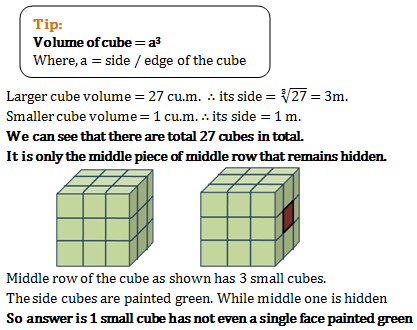4)   Paper charge is Rs. 60 per kg. How much expenditure would be there to cover a cube of edge 10m with a paper, if one kg of paper covers 20 sq.m. area?
- Published on 03 May 17

a. Rs. 2250
b. Rs. 3600
c. Rs. 2700
d. Rs. 1800
 Answer  Explanation ANSWER: Rs. 1800 Explanation: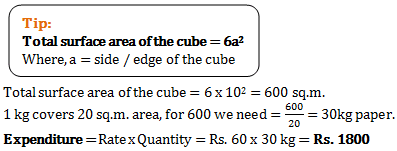5)   Sam has a solid metal ball with diameter 6cm. He melts it and uses the material for making a solid cylinder. If the diameter of the cylinder is same as the ball, what would its height be?
- Published on 03 May 17

a. 4 cm
b. 4.5 cm
c. 6 cm
d. 8 cm
 Answer  Explanation ANSWER: 4 cm Explanation: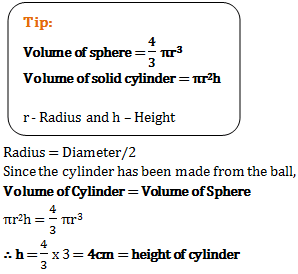6)   Raju has a cone, a hemisphere and a cylinder. They have the same height. Raju immerses them completely in a bucket full of water. What will be ratio of volume of cylinder to cone to hemisphere, if they also have same bases?
- Published on 03 May 17

a. 1:2:3
b. 3:1:2
c. 2:1:3
d. 1:1:1
 Answer  Explanation ANSWER: 3:1:2 Explanation: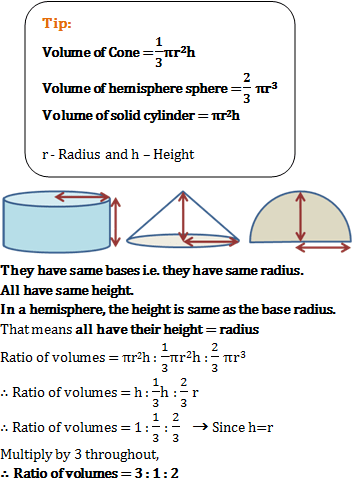7)   Ramesh has a rectangular wooden block. P, Q and R are the areas of the three adjacent and contiguous faces of the block. If we denote its volume by S, then which of the following is true for sure?
- Published on 03 May 17

a. S = 2(P+Q+R)
b. S = P+Q+R
c. S2 = PQR
d. S2 = (PQR)/2
 Answer  Explanation ANSWER: S2 = PQR Explanation: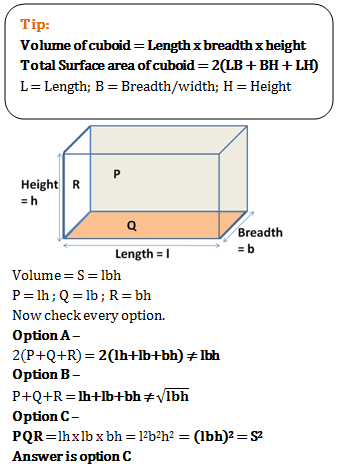8)   A steel vessel has a base of length 60 cm and breadth 30 cm. Water is poured in the vessel. A cubical steel box having edge of 30 cm is immersed completely in the vessel. How much will the water rise?
- Published on 03 May 17

a. 7.5 cm rise
b. 10 cm rise
c. 15 cm rise
d. 30 cm rise
 Answer  Explanation ANSWER: 15 cm rise Explanation: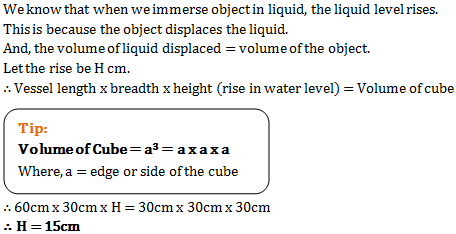9)   A cylinder having radius 1 m and height 5 m, is completely filled with wine. In how many conical flasks can this wine be filled into if the flask radius and height is 50 cm each?
- Published on 12 Apr 17

a. 50
b. 500
c. 120
d. 160
 Answer  Explanation ANSWER: 120 Explanation: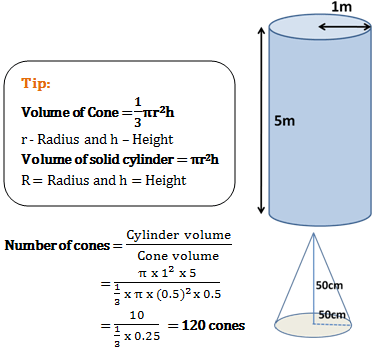10)   Ajay has 2 cones with him. 1st cone has radius twice the other and height half the other. What is volume ratio of 1st and 2nd cones?
- Published on 12 Apr 17

a. 2:1
b. 3:4
c. 1:2
d. 4:3
 Answer  Explanation ANSWER: 1:2 Explanation: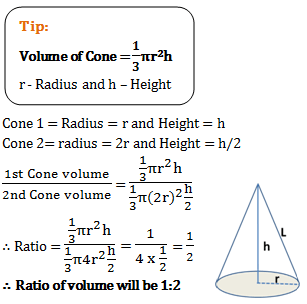1 2 3